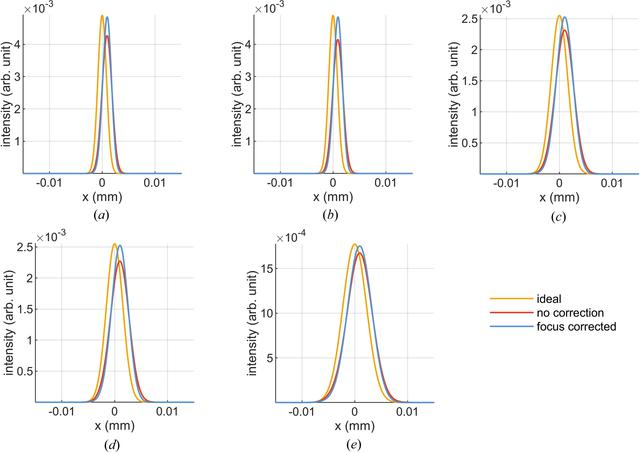disable zoom     view article Figure 11 Compared with an ideal mirror (orange curves) the intensity distribution of the thermoelastically deformed M1 (red curves) is shifted horizontally and the peak is lower; the ratio of these peak intensities has been used to calculate the Strehl ratio. By correcting the focus the peak intensity can be increased. The horizontal shift is due to the cool-down pitch of M1 and is independent of K value. Distributions are shown for (a) K = 1.5, third harmonic, linear horizontal polarization, (b) K = 1.5, third harmonic, linear vertical polarization, (c) K = 1.5, first harmonic, linear horizontal polarization, (d) K = 1.5, first harmonic, linear vertical polarization and (e) K = 2.5, first harmonic, linear horizontal polarization.JOURNAL OFSYNCHROTRONRADIATION
ISSN: 1600-5775
Volume 27| Part 5| September 2020| Pages 1131-1140